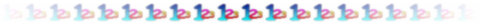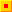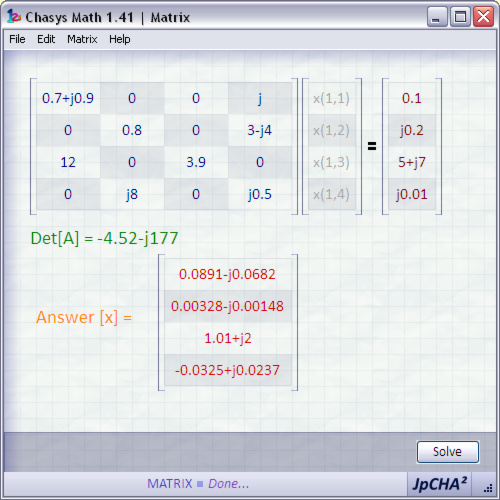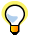...

### Chasys Math Help:Introduction to MatrixWhat is Chasys Math Matrix? Chasys Math Matrix is the matrix equation solver component of Chasys Math. It allows you to solve large systems of equations using the popular matrix method, and does so for both real and complex numbers. Systems as large as 32 by 32 variables are supported.Chasys Math Matrix uses a point-and-click interface, allowing the user to directly edit elements by clicking on them directly, which greatly enhances usability.The help file for this application is still a bit sketchy, as this is a very new app. Kindly bear with me. Thank You!The computational power of a modern computer is only adequate for matrices up to about 10x10, which takes about a second on average to calculate. 11x11 takes about 11 seconds, 12x12 takes 2 minutes, 13x13 takes 26 minutes, etc. At 32x32, the time required is 35,620,000,000,000 years!!!Created by John Paul Chacha's Lab, 2008